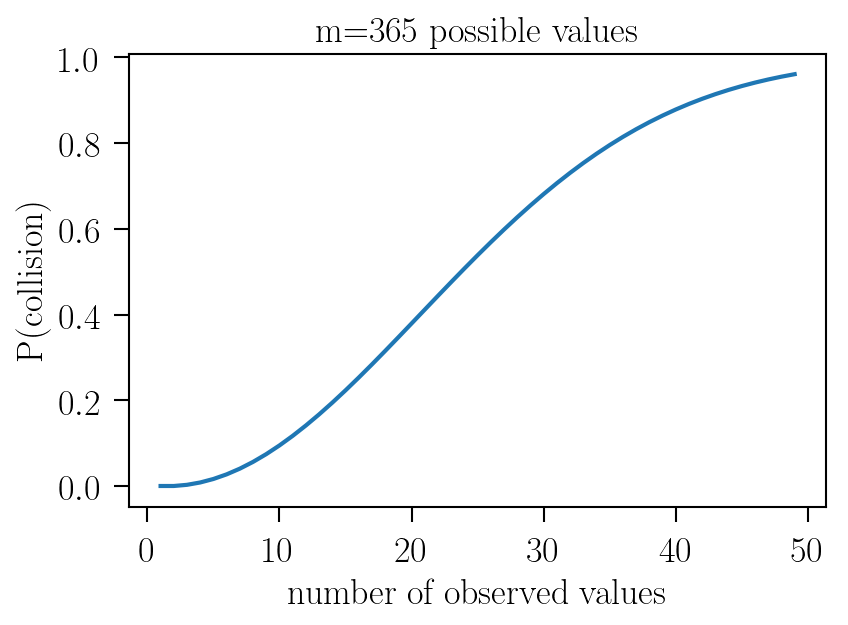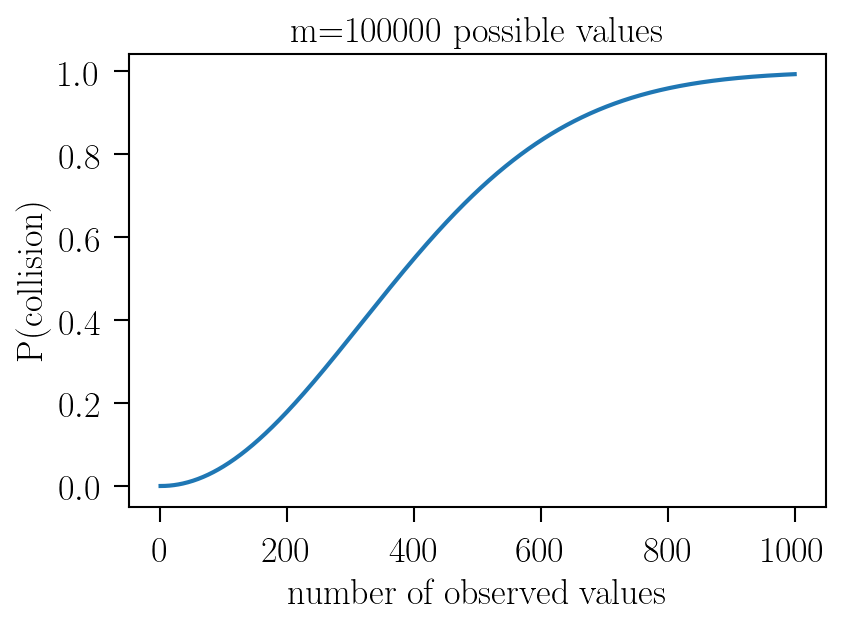# Collision attacks and the birthday paradox

How many people do you need in a room before there is a 50% chance that least two of them share the same birthday? It’s only 23, though unless you have heard about this paradox before, you might expect it to be much larger. This is the well-known birthday paradox: it’s called a paradox only because collisions happen much faster than one naively expects. Collisions here means an event where two or more observed values are equal. This paradox is important in cryptography as it’s relevant for many topics like cryptographic hash functions, which are designed to be collision-resistant one-way functions.

### The probability of collision

Let’s see why this is true by first deriving analytically the probability that no collision occurs for a generic case where there are $m$ possible values, and we observe $n$ of them. The birthday paradox above is a special case where $m=365$ and $n=23$. We want to know: what is the probability that we don’t see any collisions after $n$ observations?

We first note that the first observation, $o_1$, trivially occurs with probability 1: $P(o_1) = 1$.

Next we compute the probability that the second observation does not match the first, there are now $m-1$ possible options out of $m$:

$P(o_2 \ne o_1) = \frac{m - 1}{m} = 1 - \frac{1}{m}$

Now the third, which should not match either the first or the second:

$P(o_3 \ne o_1 \ne o_2) = \frac{m - 2}{m} = 1 - \frac{2}{m}$

And so on and so forth until the $n$th observation, which should not match any of the first $n-1$ observations:

$P(o_n \ne o_1 \ne o_2 … \ne o_{n-1}) = \frac{m - (n - 1)}{m} = 1 - \frac{n-1}{m}$

The probability of no collisions is just the joint probability of these events (assuming that they’re independent):

$P(\mbox{No collision}) = \prod_{i=1}^{n} P(o_i) = \prod_{i=1}^{n-1} 1 - \frac{i}{m}$

What is the probability that a collision does occur? Well, we know that the probability that a collision occurs is the complement of the probability that no collision occurs, i.e.:

$P(\mbox{Collision}) = 1 - P(\mbox{No collision}) = 1 - \prod_{i=1}^{n-1} 1 - \frac{i}{m}$

That is the probability for any $n$ and $m$ that a collision occurs due to the birthday paradox ✨

### Collision probability as a function of $n$

Armed with the above analytic expression, we can write a simple function that computes the probability of collision for any $n$ and $m$:

def prob_of_collision(n: int=23, m: int=365) -> int:
probability_of_no_collision = 1
for i in range(1, n - 1):
probability_of_no_collision *= 1 - i/m
return 1 - probability_of_no_collision

Let’s now use this function to make a few plots showing how the probability changes as a function of $n$.

#### Birthday case: m=365We can see that the probability reaches $50\%$ right around $n=23$, thus recovering what we stated in the introduction.

#### Larger case: m=100000For larger numbers, we see that collisions happen very fast: in a space of 100000 possible values, we reach $50\%$ probability after less than 400 observations. This should underscore the importance of considering birthday attacks especially in the case even where the space of possible values is very large. We can see empirically from these couple of cases that the number of observations $n$ you need to get a probability of collision of 0.5 is approximately $\sqrt{m}$. You can prove that to yourself analytically by taking the equation we derived above for the probability of collision, setting the left hand side equal to 0.5, and rearranging to see the relationship between $n$ and $m$.